# Examples of Solutions to Problems Involving Motion of Rigid Bodies (Part - 2) Civil Engineering (CE) Notes | EduRev

## Civil Engineering (CE) : Examples of Solutions to Problems Involving Motion of Rigid Bodies (Part - 2) Civil Engineering (CE) Notes | EduRev

The document Examples of Solutions to Problems Involving Motion of Rigid Bodies (Part - 2) Civil Engineering (CE) Notes | EduRev is a part of the Civil Engineering (CE) Course Introduction to Dynamics and Vibrations- Notes, Videos, MCQs.
All you need of Civil Engineering (CE) at this link: Civil Engineering (CE)

General discussion of slipping contacts:

Solving problems with sliding at a contact is always tricky, because we have to draw the friction forces in the correct direction.   Before tackling the example, we will summarize the general rules.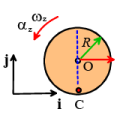We will consider a wheel as an example, but the rules apply to contact between any object and a stationary surface. The figure shows a wheel that spins with angular velocity ω = ω zk while the center moves with speed v= voxi .

The direction of the friction force is determined by the direction of motion of the point on the wheel that instantaneously touches the ground, which can be calculated from the formula

vC = (vOx + ωzR)i

Friction always acts to try to bring point C to rest – if C is moving to the right, friction acts to the left; if C is moving to the left, friction acts to the right.

There are three possible cases:

Forward slip: vOx + ω zR> 0 Point C moves in the positive i direction over the ground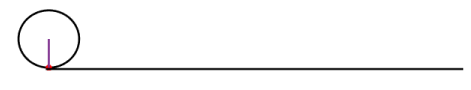• Slip occurs at the contact,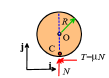• We have to use the friction law T = µN
• Point C is moving to the right, so friction must act to the left

Pure rolling vOx + ω zR= 0 .  Point C is stationary.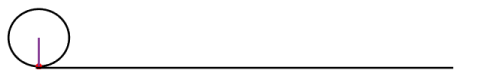• No slip occurs at the contact.
• In this case|T| < µ N
• We can draw the friction force in either direction at the contact (if we choose the wrong direction, our calculations will just tell us that T is negative).  It is usually convenient to choose T to act in the positive i direction, but this is not necessary.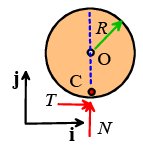Reverse slip: vOx + ωzR<0 Point C moves in the negative i direction over the ground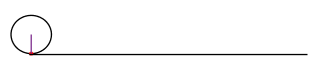• Slip occurs at the contact,
• We have to use the friction law T = µN
• Point C is moving to the left, so friction must act to the right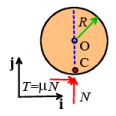4.1 Draw a free body diagram showing the forces acting on the disk just after it hits the ground.

We are given that vx0 and ωz0 are both positive so we have vOx + ωzR> 0 .  This is forward slip, and we use the corresponding FBD.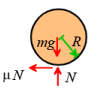4.2 Hence, find formulas for the initial acceleration a and angular acceleration α for the disk, in terms of g , R and µ .  Note that the contact point is slipping.

The equations of motion are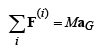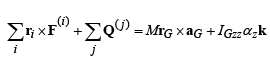-μNi + (N - mg) j = max i

- μNRk = IGzzαz k

Solving these and using IGzz = mR2 /2 :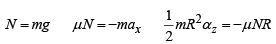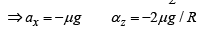4.3 Find formulas for the velocity and angular velocity of the disk, during the period while the contact point is still slipping.

The acceleration and angular acceleration are constant, so we can use the constant acceleration formulas:

vx = v0 − µgt ωz0 − 2µgt/ R

4.4 Find a formula for the time at which the disk will reverse its direction of motion.

Velocity is reversed where v=0.   From the previous part, v = v0 − µgt ⇒ t= v0 /µg at the reversal.

4.5 Find a formula for the time at which the disk begins to roll on the ground without slip.   Hence, show that the disk will reverse its direction only if v0< ω0R /2

Rolling without sliding starts when vxO = −ωzR .   We have that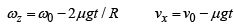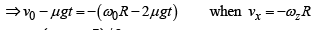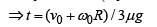The reversal will only occur if rolling without slip occurs after the reversal of velocity. This means

(v00R) /3µg >v0 /µg ⇒ v00R / 2

Example 5: The ‘Sweet Spot’ on a softball or baseball bat, or tennis or squash racket is a point that minimizes the reaction forces acting on the athlete’s hand when the ball is struck.   In fact, any rigid body has a sweet spot – the magic point is called the ‘center of percussion’ of a rigid body.

For baseball and softball bats in particular, there is a standard ASTM test that can be used to measure the position of the sweet spot.   The test works like this: the bat is suspended from the knob on handle, so it swings like a pendulum.   The period of vibration τ of the swinging bat is then measured.  ASTM say that the center of percussion is then a distance

d = π2g/4π2

from the end of the handle.   Why does this work?   It seems that this test has nothing whatever to do with a ball hitting the bat!

We will solve this problem in two parts.  First, we will calculate a formula for the period of vibration in the ASTM test.   Then we will calculate the position of the center of percussion.  We will see that the ASTM test does indeed make the correct prediction.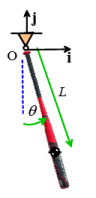We can calculate the period using the energy method.  The figure shows the ASTM pendulum test.  We assume that

• The bat has a mass moment of inertia about its COM IGzz
• The COM is a distance L from O

The bat pivots about O, so we can use the fixed axis rotation formula for the kinetic energy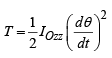Here IOzz = IGzz + ML(using the parallel axis theorem).

The potential energy is V = − MgL cosθ

Energy conservation gives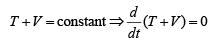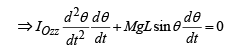If θ is small then sinθ ≈θ so the equation of motion reduces to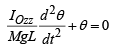This is a standard ‘Case 1’ EOM.  The natural frequency is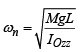so the period is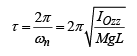Next, we find the position of the ‘sweet spot’. We can do this by calculating the reaction forces on the handle when the bat is struck, and finding the impact point that minimizes the reaction force.

The figure shows an impact event.   We assume that:

• The bat rotates in the horizontal plane (so gravity acts out of the plane of the figure).
• The bat rotates about the point O
• The ball impacts the bat a distance d from the handle.
• The ball exerts a (large) force Fimpact on the bat
• Reaction forces Rx ,Ry act on the handle during the impact.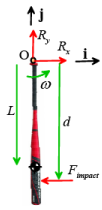This is a planar problem, so we can use the 2D equations of motion. The equation for translational motion gives

( R−Fimpact)i + Ry j= MaG

For the rotational equation we can also use the short-cut for fixed axis rotation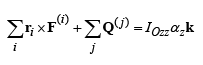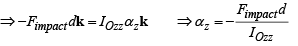We can relate ato αz using the rigid body formula:

aG zk ×rG−ω2 z rG =αz Li +ω2 zLj

We therefore see that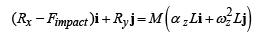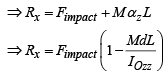The sweet spot is at the position that makes Rx = 0 , which shows that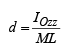For comparison, the ASTM formula gives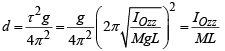Offer running on EduRev: Apply code STAYHOME200 to get INR 200 off on our premium plan EduRev Infinity!

## Introduction to Dynamics and Vibrations- Notes, Videos, MCQs

20 videos|53 docs

,

,

,

,

,

,

,

,

,

,

,

,

,

,

,

,

,

,

,

,

,

;